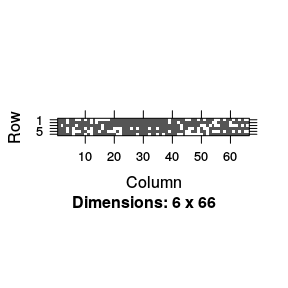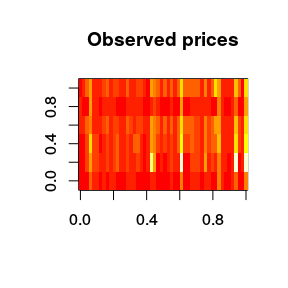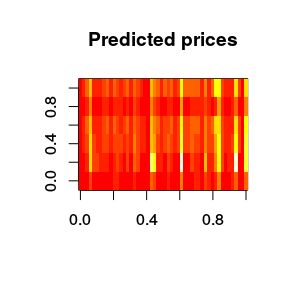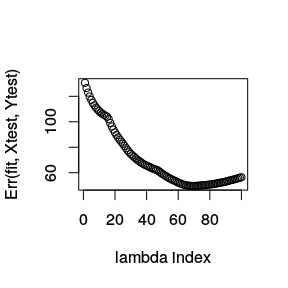# Quick Start

## Quick Start (for lsgl version 1.3.5)

### 1. Load the msgl library in R

library(lsgl)
## Loading required package: Matrix
## Loading required package: sglOptim
## Loading required package: foreach
## Loading required package: doParallel
## Loading required package: iterators
## Loading required package: parallel

Load data containing N samples and p features (covariates) and a response matrix containing K responses for each sample:

X <- # load design matrix (of size N x p)
Y <- # load response matrix (of size N x K)

For the purpose of this tutorial we will load a data set consisting of airline ticket prices

data(AirlineTicketPrices)
dim(X)
##  337 411
dim(Y)
##  337   6

Hence, p = 411, N = 337 and the dimension of the response K = 6, this implies that the model has 6*(411+1) = 2472 parameters.

Let us take out a small test set:

idx <- sample(1:nrow(X), size = 50)

Xtest <- X[idx, ]
Ytest <- Y[idx, ]
X <- X[-idx, ]
Y <- Y[-idx, ]

### 3. Estimate error using cross validation

Choose lambda (fraction of lambda.max) and alpha, with alpha = 1 for lasso, alpha = 0 for group lasso and alpha in the range (0,1) for spares group lasso.

Use lsgl::cv to estimate the error for each lambda in a sequence decreasing from the data derived lambda max to lambda * lambda max. Lambda max is the lambda at which the first penalized parameter becomes non-zero. A smaller lambda will take longer to fit and include more features. The following command will run a 10 fold cross validation for each lambda value in the lambda sequence using 2 parallel units (using the foreach and doParallel packages.

cl <- makeCluster(2)
registerDoParallel(cl)

# Do cross validation -- this may take some time
fit.cv <- lsgl::cv(X, Y, fold = 10, alpha = 0.5, lambda = 0.001, use_parallel = TRUE)
##
## Running lsgl 10 fold cross validation
##
##  Samples:  Features:  Models:  Groups:  Parameters:
##        287        412        6      412       2.472k
stopCluster(cl)

(for the current version no progress bar will be shown)

Get a summery of the validated models. We have now cross validated the models corresponding to the lambda values, one model for each lambda value. We may get a summery of this validation by doing:

fit.cv
##
## Call:
## lsgl::cv(x = X, y = Y, alpha = 0.5, lambda = 0.001, fold = 10,
##     use_parallel = TRUE)
##
## Models:
##
##  Index:  Lambda:  Features:  Parameters:  Error:
##        1    1.000          1            6     132
##       20    0.266        6.8         40.8      91
##       40    0.066       14.3         81.7      68
##       60    0.016       38.9        178.7      57
##       80    0.004       75.2        327.3      53
##      100    0.001      167.9        780.4      59
##
## Best model:
##
##  Index:  Lambda:  Features:  Parameters:  Error:
##       78   0.0046       69.7        304.6      53

Hence, the best model is obtained using lambda index 78 and it has a cross validation error of 52.58. The expected number of selected features is 69.7 and the expected number of parameters is 304.6.

### 4. Fit the final model

Use lsgl to fit a final model.

fit <- lsgl::fit(X, Y, alpha = 0.5, lambda = 0.001)
##
## Running lsgl
##
##  Samples:  Features:  Models:  Groups:  Parameters:
##        287        412        6      412       2.472k

Get a summery of the estimated models

fit
##
## Call:
## lsgl::fit(x = X, y = Y, alpha = 0.5, lambda = 0.001)
##
## Models:
##
##  Index:  Lambda:  Features:  Parameters:
##        1    1.000          1            6
##       20    0.266          7           42
##       40    0.066         13           75
##       60    0.016         41          185
##       80    0.004         72          318
##      100    0.001        168          781

Take a look at the estimated models. As we saw in the previous step the model with index 78 had the best cross validation error, we may take a look at the included features using the command:

features(fit)[[best_model(fit.cv)]][1:10] # Ten first non-zero features in best model
##   ""                  "'ALLminpA'"        "'ALLnumquotesA'l1"
##   "'ALLnumquotesA'l2" "'ALLnumquotesA'l7" "'ALLminp0'"
##   "'ALLminp0'l1"      "'ALLnumquotes2'"   "'ALLnumquotes4'"
##  "'ALLnumquotes4'l2"

Hence 66 features are included in the model, this is close to the expected number based on the cross validation estimate.

The sparsity structure of the parameters belonging to these 66 features may be viewed using

image(parameters(fit)[[best_model(fit.cv)]])We may also take a look at the estimate parameters (or coefficients)

coef(fit, best_model(fit.cv))[,1:5] # First 5 non-zero parameters of best model
## 6 x 5 sparse Matrix of class "dgCMatrix"
##                                  'ALLminpA' 'ALLnumquotesA'l1
## LBL+ALLminpA+fut_001   17.71382  0.20820377       0.008067314
## LBL+ALLminp0+fut_001 -187.54631  0.01153876       0.035593658
## LBL+aCOminpA+fut_001 -133.83585 -0.00638412       0.020849783
## LBL+aFLminpA+fut_001  -15.95476  0.10929823       0.006593796
## LBL+aUAminpA+fut_001 -158.03778 -0.03329178       0.028548041
##                      'ALLnumquotesA'l2 'ALLnumquotesA'l7
## LBL+ALLminpA+fut_001      .                 -0.008652889
## LBL+ALLminp0+fut_001      .                 -0.029042608
## LBL+aCOminpA+fut_001      .                 -0.002412663
## LBL+aFLminpA+fut_001      .                 -0.011981335
## LBL+aUAminpA+fut_001     -4.938302e-06      -0.002105731

If we count the total number of non-zero parameters in the model we get, in this case 292 which is close to the expected based on the cross validation estimate.

### 6. Use your model for predictions

Load test data containing M samples and p features.

Xtest <- # load matrix with test data (of size M x p)

Use the final model to predict the price vector of the M=50 samples in Xtest.

res <- predict(fit, Xtest)

Plot predicted and true response

image(Ytest, main = "Observed prices")image(res\$Yhat[[best_model(fit.cv)]], main = "Predicted prices")Compute the error rates on the test set

plot(Err(fit, Xtest, Ytest), xlab = "lambda index")